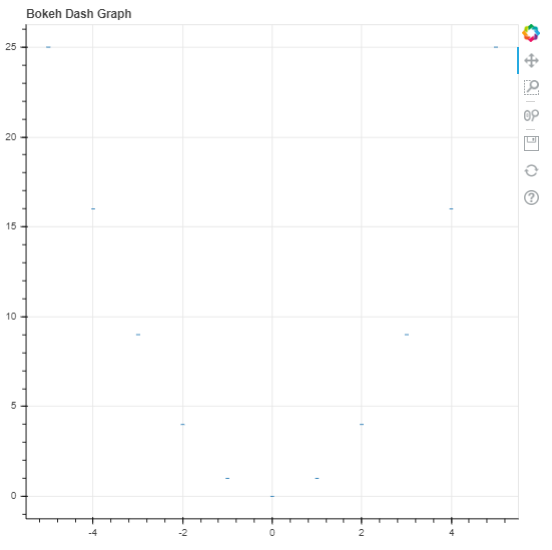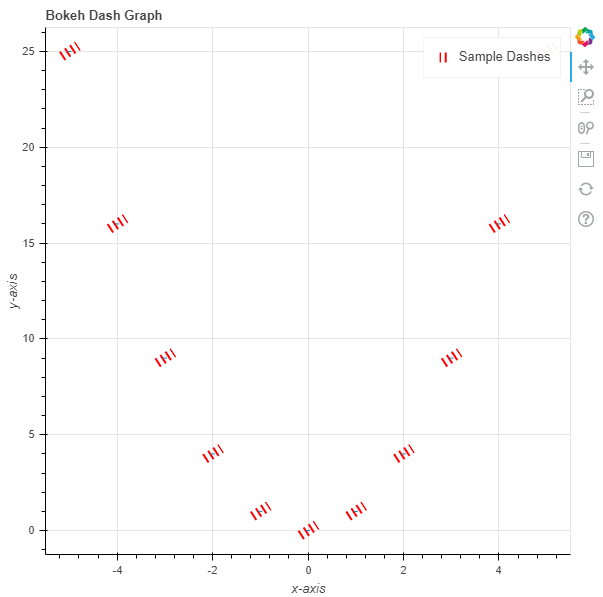GeeksforGeeks App
Open AppBrowser
Continue

# Python Bokeh – Plotting Dashes on a Graph

Bokeh is a Python interactive data visualization. It renders its plots using HTML and JavaScript. It targets modern web browsers for presentation providing elegant, concise construction of novel graphics with high-performance interactivity.

Bokeh can be used to plot dashes on a graph. Plotting dashes on a graph can be done using the `dash()` method of the `plotting` module.

## plotting.figure.dash()

Syntax : dash(parameters)

Parameters :

• x : x-coordinates of the center of the dash markers
• y : y-coordinates of the center of the dash markers
• size : diameter of the dash markers, default is 4
• angle : angle of rotation of the dash markers, default is 0
• angle_units : unit of the angle, default is rad
• fill_alpha : fill alpha value of the dash markers
• fill_color : fill color value of the dash markers
• line_alpha : percentage value of line alpha, default is 1
• line_cap : value of line cap for the line, default is butt
• line_color : color of the line, default is black
• line_dash : value of line dash such as :
• solid
• dashed
• dotted
• dotdash
• dashdot

default is solid

• line_dash_offset : value of line dash offset, default is 0
• line_join : value of line join, default in bevel
• line_width : value of the width of the line, default is 1
• name : user-supplied name for the model
• tags : user-supplied values for the model

Other Parameters :

• alpha : sets all alpha keyword arguments at once
• color : sets all color keyword arguments at once
• legend_field : name of a column in the data source that should be used
• legend_group : name of a column in the data source that should be used
• legend_label : labels the legend entry
• muted : determines whether the glyph should be rendered as muted or not, default is False
• name : optional user-supplied name to attach to the renderer
• source : user-supplied data source
• view : view for filtering the data source
• visible : determines whether the glyph should be rendered or not, default is True
• x_range_name : name of an extra range to use for mapping x-coordinates
• y_range_name : name of an extra range to use for mapping y-coordinates
• level : specifies the render level order for this glyph

Returns : an object of class `GlyphRenderer`

Example 1 :In this example we will be using the default values for plotting the graph.

 `# importing the modules``from` `bokeh.plotting ``import` `figure, output_file, show``   ` `# file to save the model``output_file(``"gfg.html"``)``   ` `# instantiating the figure object``graph ``=` `figure(title ``=` `"Bokeh Dash Graph"``)``  ` `# the points to be plotted``x ``=` `[``-``5``, ``-``4``, ``-``3``, ``-``2``, ``-``1``, ``0``, ``1``, ``2``, ``3``, ``4``, ``5``]``y ``=` `[``25``, ``16``, ``9``, ``4``, ``1``, ``0``, ``1``, ``4``, ``9``, ``16``, ``25``]``   ` `# plotting the dashes``graph.dash(x, y)``   ` `# displaying the model``show(graph)`

Output :Example 2 :In this example we will be plotting the dashes with dotted lines alongside other parameters.

 `# importing the modules``from` `bokeh.plotting ``import` `figure, output_file, show``   ` `# file to save the model``output_file(``"gfg.html"``)``   ` `# instantiating the figure object``graph ``=` `figure(title ``=` `"Bokeh Dash Graph"``)`` ` `# name of the x-axis``graph.xaxis.axis_label ``=` `"x-axis"``  ` `# name of the y-axis``graph.yaxis.axis_label ``=` `"y-axis"`` ` `# the points to be plotted``x ``=` `[``-``5``, ``-``4``, ``-``3``, ``-``2``, ``-``1``, ``0``, ``1``, ``2``, ``3``, ``4``, ``5``]``y ``=` `[``25``, ``16``, ``9``, ``4``, ``1``, ``0``, ``1``, ``4``, ``9``, ``16``, ``25``]`` ` `# size of the dashes``size ``=` `20`` ` `# angle of the dashes``angle ``=` `10`` ` `# color of the line``line_color ``=` `"red"``  ` `# type of line``line_dash ``=` `"dotted"``  ` `# offset of line dash``line_dash_offset ``=` `1`` ` `# width of the dashes``line_width ``=` `10`` ` `# name of the legend``legend_label ``=` `"Sample Dashes"``  ` `# plotting the line graph for AAPL``graph.dash(x, y,``           ``size ``=` `size,``           ``angle ``=` `angle,``           ``line_color ``=` `line_color,``           ``line_dash ``=` `line_dash,``           ``line_dash_offset ``=` `line_dash_offset,``           ``line_width ``=` `line_width,``           ``legend_label ``=` `legend_label)``   ` `# plotting the dashes``graph.dash(x, y)``   ` `# displaying the model``show(graph)`

Output :My Personal Notes arrow_drop_up